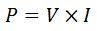# Electric Power

Definition: The rate at which the work is being done in an electrical circuit is called an electric power. In other words, the electric power is defined as the rate of the transferred of energy. The electric power is produced by the generator and can also be supplied by the electrical batteries. It gives a low entropy form of energy which is carried over long distance and also it is converted into various other forms of energy like motion, heat energy, etc.

The electric power is divided into two types, i.e., the AC power and the DC power. The classification of the electric power depends on the nature of the current. The electric power is sold regarding joule which is the product of the power in kilowatts and the running time of the machinery in hours. The utility of power is measured by the electric meter which records the total energy consumed by the powered devices. The electric power is given by the equation shown below.Where V is the voltage in volts, I is the current in amperes, R is the resistance offered by the powered devices, T is the time in seconds and the P is the power measured in watts.

## Unit of Electric Power

The unit of electrical power is Watt.

If,Thus, the power consumed in an electrical circuit is said to one watt if one ampere current flows through the circuit when a potential difference of 1 volt is applied across it.The bigger unit of electrical power is the kilowatt (kW), it is usually used in the power system## Types of an Electric Power

The electrical power is mainly classified into two types. They are the DC power and the AC power.

## 1. DC Power

The DC power is defined as the product of the voltage and current. It is produced by the fuel cell, battery and generator.Where P – Power in watt.
V – voltage in volts.
I – current in amps.

## 2. AC Power

The AC power is mainly classified into three types. They are the apparent power, active power and real power.

1. Apparent Power – The apparent power is the useless power or idle power. It is represented by the symbol S, and their SI unit is volt-amp.Where S – apparent power
Vrms – RMS voltage = Vpeak√2 in volt.
Irms – RMS current = Ipeak√2 in the amp.

2. Active Power – The active power (P) is the real power which is dissipated in the circuit resistance.Where, P – the real power in watts.
Vrms – RMS voltage = Vpeak√2 in volts.
Irms – RMS current = Ipeak√2 in the amp.
Φ – impedance phase angle between voltage and current.

3. Reactive Power – The power developed in the circuit reactance is called reactive power (Q). It is measured in volt-ampere reactive.Where, Q – the reactive power in watts.
Vrms – RMS voltage = Vpeak√2 in volt.
Irms – RMS current = Ipeak√2 in the amp.
Φ – impedance phase angle between voltage and current.

The relation between the apparent, active and reactive power is shown below.The ratio of the real to the apparent power is called power factor, and their value lies between 0 and 1.